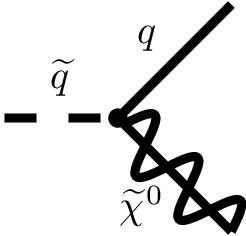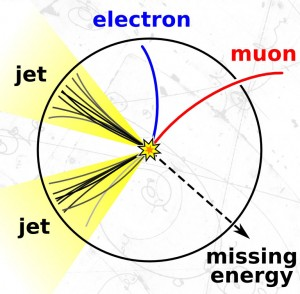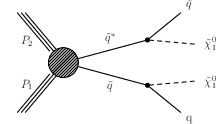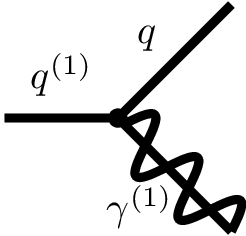Categories
Pages
-Simplified models for new physics

February 13th, 2015 | by

Jan Heisig and Jory Sonneveld report on their recent work on simplified models

With about a petabyte of data processed in Switzerland everyday, the Large Hadron Collider (LHC) provides an enormous amount of information on high energy physics processes. This information is used in order to test theories beyond the Standard Model of Particles Physics — theories that are motivated either by outstanding theoretical problems or experimental evidence, like in the case of dark matter. While experimentalists work their way through the data, theorists line up to convince them to search for their favorite a model in the currently collected 20 fb-1 of data.It is impossible for experimentalists to search for each possible model theorists came up with. This is why they often try to search for simple characteristics that represent a larger class of possible new models of physics. One new model of physics, supersymmetry, for example, predicts new spin-0 (scalar) quarks, or squarks (supersymmetric quarks) among many other particles. These new squarks decay to a quark and so-called neutralino (see Feynman diagram on the left), which in many models of supersymmetry is assumed to be the lightest supersymmetric particle.From FNAL.gov

What would be seen at the LHC if supersymmetry were realized in nature? As the neutralino is a neutral, stable particle it is invisible for the detector. But as it carries away energy and momentum it could be reconstructed with the missing energy in an event. However, in order to recognize that energy is missing we have to measure visible particles the neutralino recoils against. If at the LHC a pair of squarks is produced in the collision of two protons and both squarks decay in a quarks and a neutralino, we would see events with two quarks (recognized as “jets” in the detector) and missing energy from the invisible neutralinos. This is an important signature that is looked for at the LHC.

How could we interpret the presence or absence of a signal in the search for jets and missing energy in a specific model? This is not trivial, since the significance of the search in general depends on details of the model. For instance, as supersymmetry has a huge number of free parameters, the significance of the search can in principle depend on the masses of supersymmetric particles other than the squark and neutralino.In this article we investigated this question studying to what extent the “simplified squark model” (left figure) introduced by the experimental collaborations can be used to draw conclusions about more general supersymmetric models where the production is also mediated by a gluino, the supersymmetric partner of the gluon (as shown in the Feynman diagram on the right).

In addition to supersymmetry, there are many other possible models of physics beyond the Standard Model.One such model postulates extra spatial dimensions (see also notes by various speakers at the TASI Lectures). It also predicts new quarks (see Feynman diagram on the right), but this time particles with spin 1/2: this means they have the same spin as the Standard Model quarks. We can call this model a same-spin model. Could we also use the results for a supersymmetric simplified squark model to say something about excluded masses of quarks and the lightest particles of a same-spin model? It turns out that one can.

We theorists then continue to use results from searches for simplified models and apply these to our favorite models of physics beyond the Standard Model. Many tools exist for exactly this purpose; one example is SModelS. We look forward to the fresh start of the LHC this year!